Ex 4.2

Chapter 4 Class 10 Quadratic Equations
Serial order wise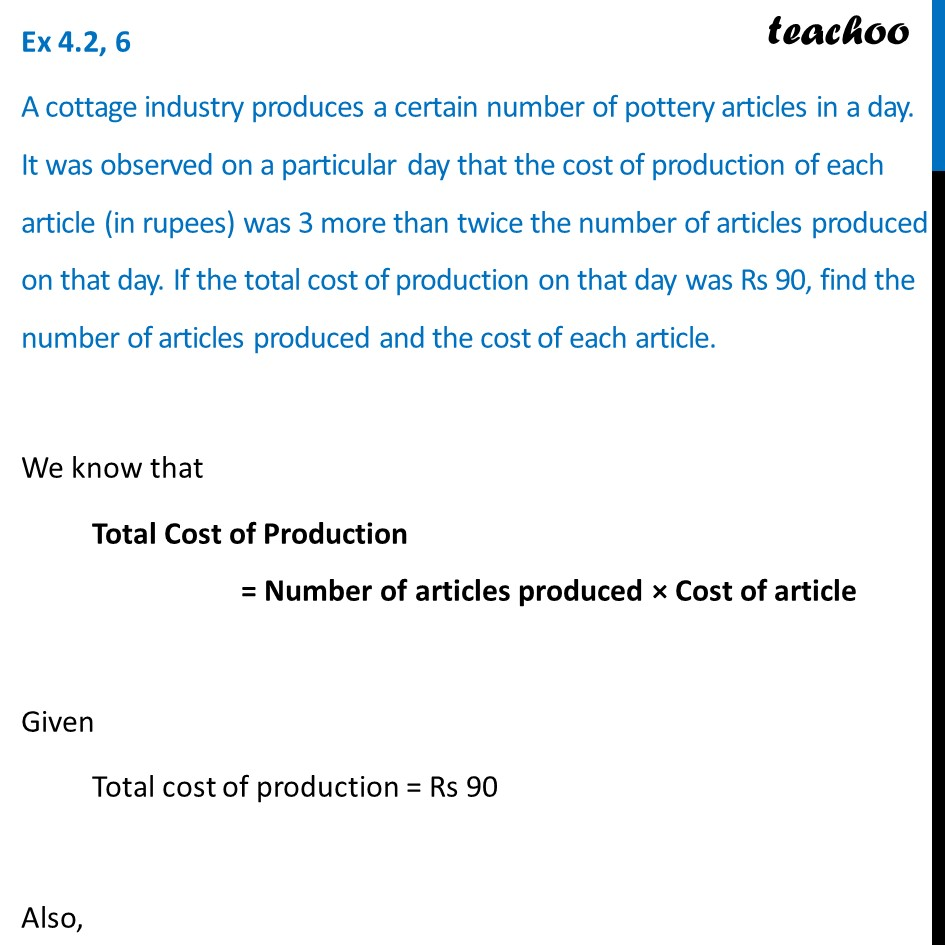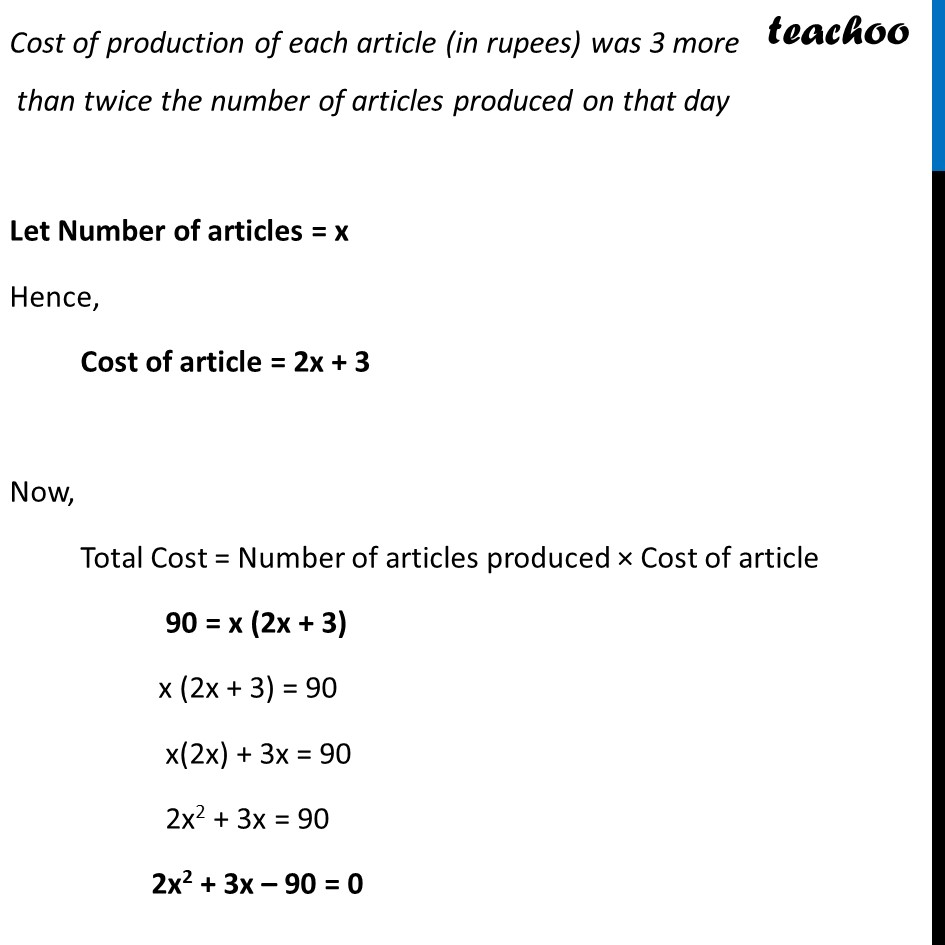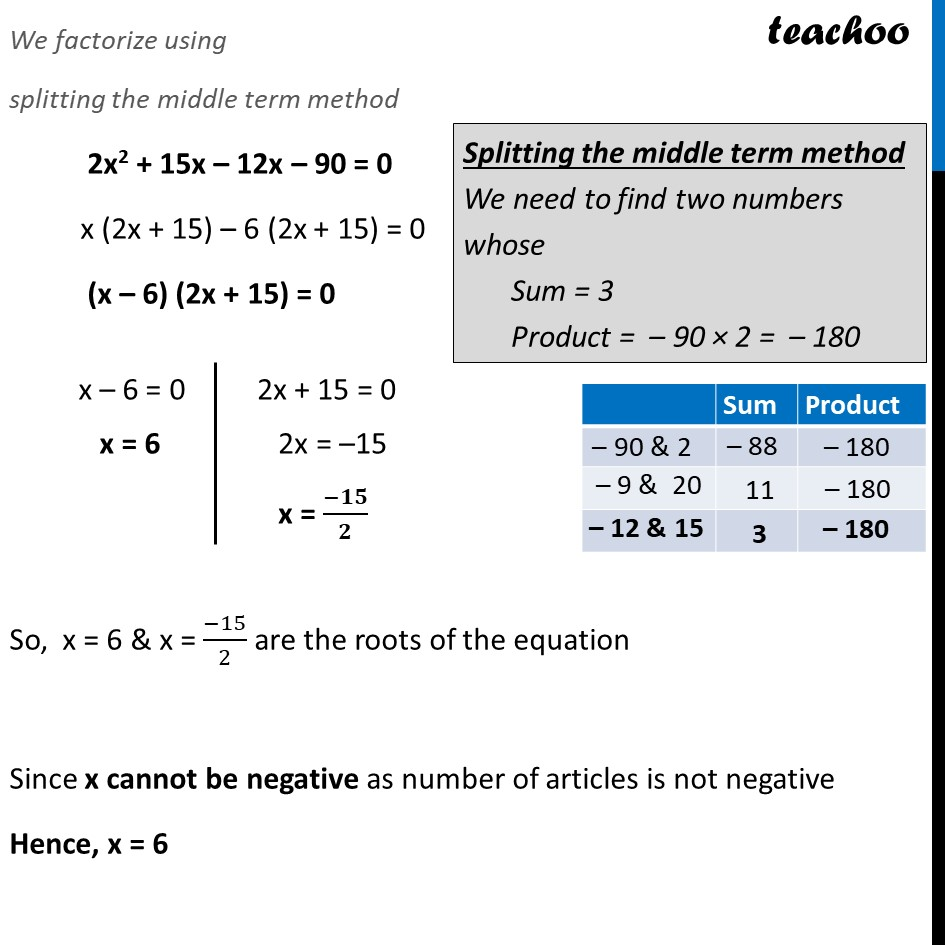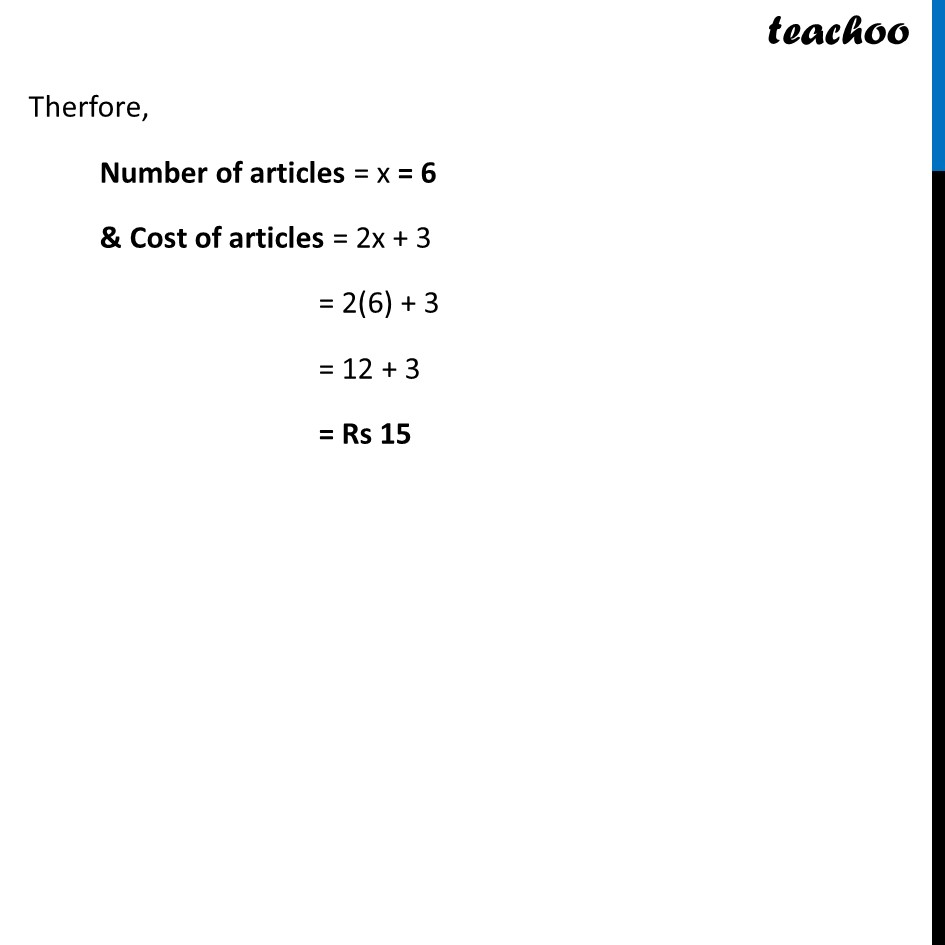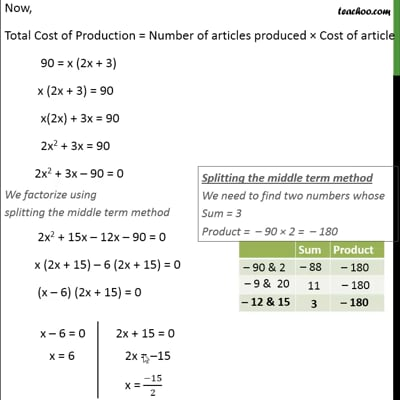This video is only available for Teachoo black users

Learn in your speed, with individual attention - Teachoo Maths 1-on-1 Class

### Transcript

Ex 4.2, 6 A cottage industry produces a certain number of pottery articles in a day. It was observed on a particular day that the cost of production of each article (in rupees) was 3 more than twice the number of articles produced on that day. If the total cost of production on that day was Rs 90, find the number of articles produced and the cost of each article. We know that Total Cost of Production = Number of articles produced × Cost of article Given Total cost of production = Rs 90 Also, Cost of production of each article (in rupees) was 3 more than twice the number of articles produced on that day Let Number of articles = x Hence, Cost of article = 2x + 3 Now, Total Cost = Number of articles produced × Cost of article 90 = x (2x + 3) x (2x + 3) = 90 x(2x) + 3x = 90 2x2 + 3x = 90 2x2 + 3x – 90 = 0 We factorize using splitting the middle term method 2x2 + 15x – 12x – 90 = 0 x (2x + 15) – 6 (2x + 15) = 0 (x – 6) (2x + 15) = 0 So, x = 6 & x = (−15)/2 are the roots of the equation Since x cannot be negative as number of articles is not negative Hence, x = 6 x – 6 = 0 x = 6 2x + 15 = 0 2x = –15 x = (−𝟏𝟓)/𝟐 Therfore, Number of articles = x = 6 & Cost of articles = 2x + 3 = 2(6) + 3 = 12 + 3 = Rs 15